Total marks: --
Total time: --
INSTRUCTIONS
(1) Assume appropriate data and state your reasons
(2) Marks are given to the right of every question
(3) Draw neat diagrams wherever necessary

Answer any one question from Q1 and Q2
1 (a) Calculate the current I through 6Ω resistor by applying principle of superposition.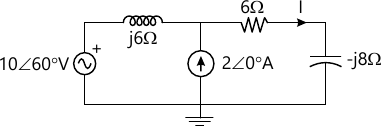6 M
1 (b) The reduced incidence matrix of an oriented graph is: $A=\begin{bmatrix} 0 &-1 &1 &0 &0 \\0 &0 &-1 &-1 &-1 \\-1 &0 &0 &0 &1 \end{bmatrix}$ Draw the oriented graph. Also calculate the number of trees possible for this graph.
6 M

2 (a) Find the value of load impedance ZL, so that maximum power can be transferred to it in the circuit shown below. Draw Thevenin's equivalent circuit.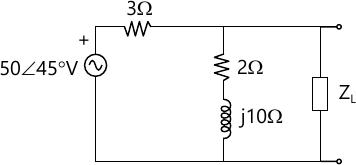6 M
2 (b) Write the incidence matrix, tieset matrix and f-cutset matrix for the graph of a network given below. Select tree {4, 5, 6}.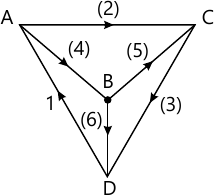6 M

Answer any one question from Q3 and Q4
3 (a) For the network shown below, find the current expression i.e. i(t) when the switch is changed from the position 1 to 2 at t = 0.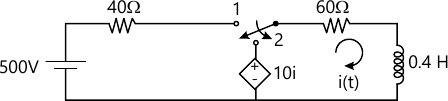6 M
3 (b) In a series RLC circuit, a maximum current of 0.1 A flows through the circuit when the capacitor is of 5 ?F with a fixed frequency and a voltage of 5 V. Determine the frequency at which the circuit resonates, the quality factor, the value of resistance and the bandwidth.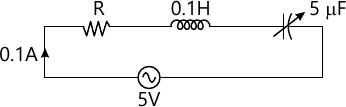6 M

4 (a) In the figure given below, the switch is closed at t = 0. Find:
$i, \dfrac {di}{dt} \ and \ \dfrac {d^2i}{dt^2} \ at \ t=o^+$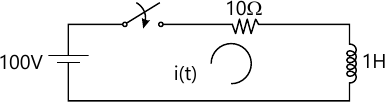6 M
4 (b) Derive the expression for bandwidth of a series RLC resonance circuit.
6 M

Answer any one question from Q5 and Q6
5 (a) Explain the following terms used in filter theory:
(i) Attenuation constant,
(ii) Phase constant, and
(iii) Characteristic impedance
6 M
5 (b) Write a short note on attenuators. Also derive the relation between decibel and Neper.
7 M

6 (a) A π section filter network consists of a series arm inductor of 20 mH and two shunt-arm capacitors of 0.16 μF each. Calculate the cut-off frequency, attenuation and phase shift at 15 kHz.
6 M
6 (b) Design a T-section band-pass, constant K type filter with cut- off frequency of 4 kHz and 10 kHz and nominal characteristic impedance of 500 Ω.
7 M

Answer any one question from Q7 and Q8
7 (a) Find Y-parameters of the network shown below: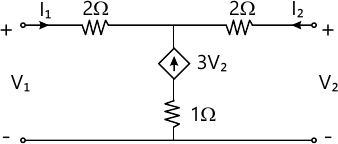6 M
7 (b) Explain the following:
i) Network functions for one and two port networks.
ii) Pole-zeros of network functions. Also state its significance.
7 M

8 (a) Calculate the open-circuit impedance parameters for the network shown below and also check for symmetry and reciprocity of the network.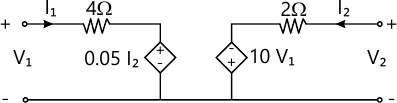6 M
8 (b) Determine the voltage transfer function $\dfrac {V_2}{V_1},$ for the network shown below.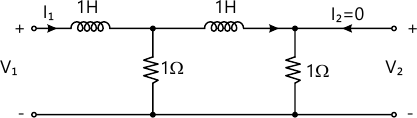7 M

More question papers from Network Theory